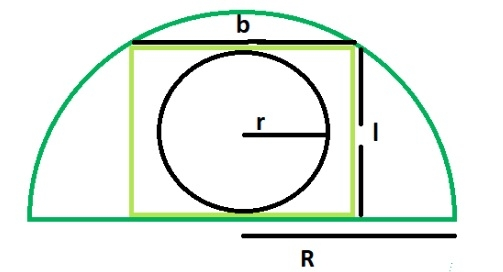# Area of a circle inscribed in a rectangle which is inscribed in a semicircle in C?

A circle inscribed in a rectangle touches the larger side of the rectangle with its ends i.e. the length is tangent to the circle.

A rectangle inscribed in a semicircle touches its arc at two points. The breadth of the rectangle is equal to the diameter of the circle.If R is the radius of semi-circle.

Length of the rectangle = √2R/2

Breadth of the rectangle = R/√2

Radius of biggest circle inscribed is

r = b/2 = R/2√2

Using this formula we can find the area of this circle inscribed in a rectangle which is inscribed in a semicircle,

Area = (π*r2) = π*R/8

## Example

Live Demo

#include <stdio.h>
int main() {
float a = 5;
float area = 3.14 * a/ 8;
printf("The area of the circle inscribed in a rectangle inscribed in a semicircle of radius %f is %f", a , area);
return 0;
}

## Output

The area of the circle inscribed in a rectangle inscribed in a semicircle of radius 5.00000 is 1.962500

Updated on: 03-Oct-2019

920 Views